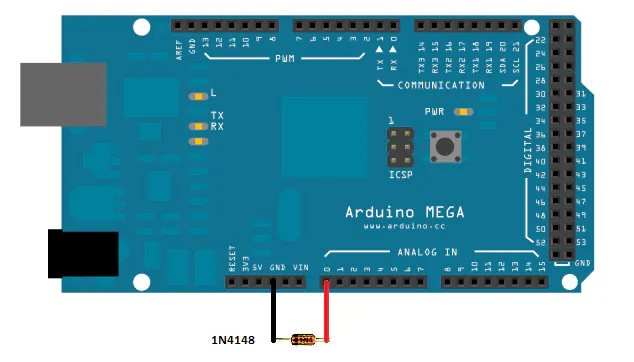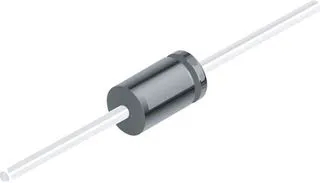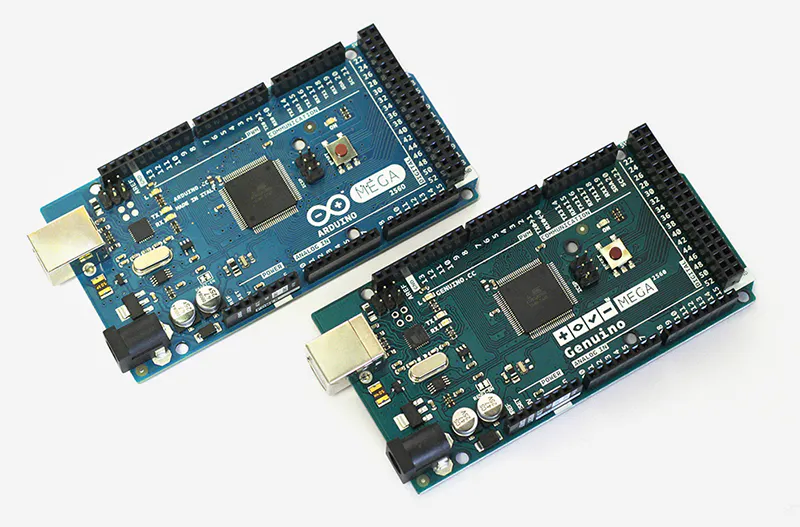# thermometer diode based

How build a simple thermometer using a silicon diode

BeginnerProtip12 minutes7,473## Things used in this project

### Hardware components1N4148 – General Purpose Fast Switching
×1Arduino Mega 2560 & Genuino Mega 2560
×1

## Schematics

### electric scheme

The scheme is very simple: It is needed to connect the diode directly to the pins of the Arduino board: In particular connect the anode at the pin A0 and the catode at the GND pin## Code

### Diode thermometer based code

Arduino
Load this code in your arduino and in the serial plot you can see the temperature read uy the diode
```/*

Thermometer based on 1n4148 silicon diode used as temperature sensor.The thermometer is based on the diode characteristic that the increase of the temperature its forward voltage (VF) is lowered by 2,2mV / ° C.
Fixing the value of Vf = VF0 at ambient temperature t0, the temperature value
t is calculated with the following formula:

t= t0 - [vf(t)- vf0]* K

with K = 1 / 2,2mV

The value of Vf (t) = dtemp -vf0 is obtained by averaging values of 1024 by acquiring as many vf values

The result of t is shown on the serial monitor
*/

// set pin numbers:

const int in = A0;          // used to bias the diode  anode
const int t0 = 20.3;
const float vf0 = 573.44;
// variables will change:

int i;
float dtemp, dtemp_avg, t;

void setup() {
Serial.begin(9600);
pinMode(in, INPUT_PULLUP);            // set the  pin IN with npull up to bias the diode

}

void loop() {
dtemp_avg = 0;
for (i = 0; i < 1024; i++) {
float vf = analogRead(A0) * (4976.30 / 1023.000);
//Serial.println(vf);
dtemp = (vf - vf0) * 0.4545454;
dtemp_avg = dtemp_avg + dtemp;
}
t = t0 - dtemp_avg / 1024;
Serial.print("temperature in Celcius degree)   = " );
Serial.println(t);

delay (1000);

}
```

## Credits

### microst

9 projects • 7 followers
Electronic is my life passion...